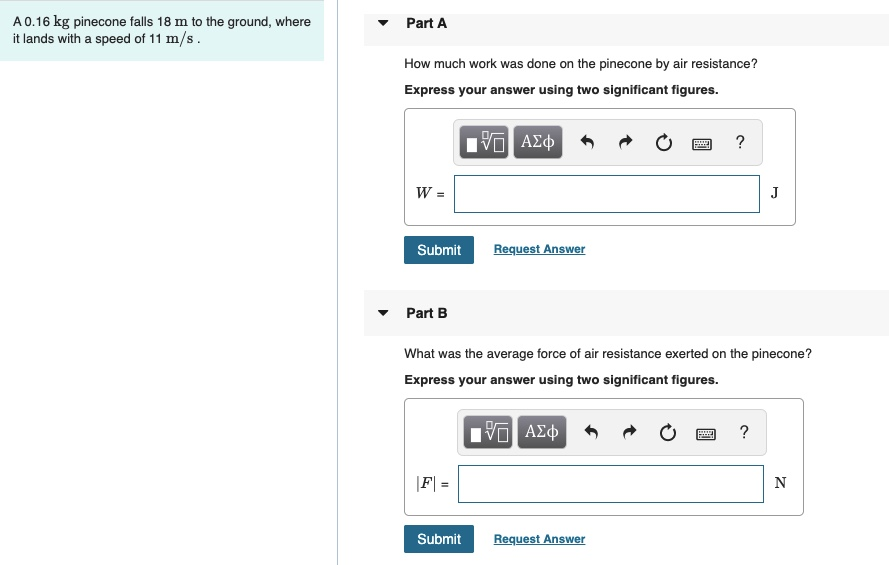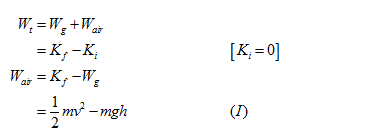# A0.16 kg pinecone falls 18 m to the ground, whereit lands with a speed of 11 m/s.Part AHow much work was done on the pinecone by air resistance?Express your answer using two significant figures.Πν ΑΣφJSubmitRequest AnswerPart BWhat was the average force of air resistance exerted on the pinecone?Express your answer using two significant figures.Hνα ΑΣφ|F| =SubmitRequest Answer

Question
4 viewshelp_outlineImage TranscriptioncloseA0.16 kg pinecone falls 18 m to the ground, where it lands with a speed of 11 m/s. Part A How much work was done on the pinecone by air resistance? Express your answer using two significant figures. Πν ΑΣφ J Submit Request Answer Part B What was the average force of air resistance exerted on the pinecone? Express your answer using two significant figures. Hνα ΑΣφ |F| = Submit Request Answer fullscreen
check_circle

Step 1

(A)

The work done on pinecone by air resistance is the difference in kinetic energies between pinecone under the influence of air resistance and not under air resistance.

Step 2

The total work done is equal to the work done due to gravity and work done by air resistance; according to work energy theorem the work done is equal to the change in kinetic energy.Substitute the values in equation (I)....

### Want to see the full answer?

See Solution

#### Want to see this answer and more?

Solutions are written by subject experts who are available 24/7. Questions are typically answered within 1 hour.*

See Solution
*Response times may vary by subject and question.
Tagged in